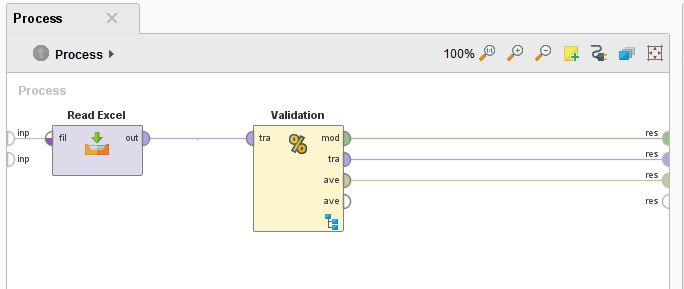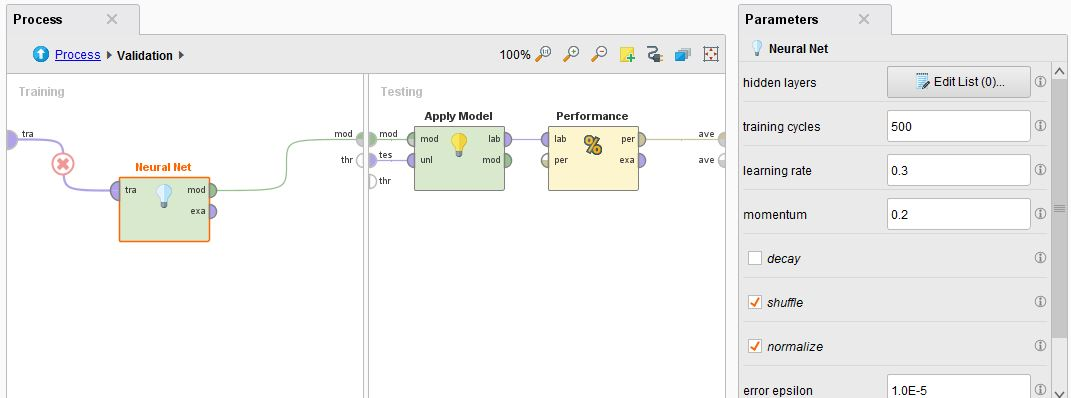# How can I debug SSE value during run Neural Net model of each loop in Rapid Miner

Member Posts: 2Contributor I
edited November 2018 in Help

Hello,

I'm student and interested to use Rapid miner run Neural Network Model to come out the formula to predict the defect using in HDD factory. I've run NN and comeout both prediction model and their accuracy using x-validation. But, I also would like to get the SSE (sum of square error) of each loop for debug more information. Any possible way to get SSE of training cycle? In this case, I set maximum 500 training cycle. Need your help. It is very critical for me..

Here are my setting picture.

Overall connection.Inside validation• RapidMiner Certified Analyst, RapidMiner Certified Expert, Member Posts: 2,531Unicorn
Solution Accepted

Hi,

you cannot get that directly, but if you use Performance (Regression) operator inside the validation you will get many more choices of how to describe the goodness of a model. One of them is squared error, which will give you the average squared error. From that (Mikro average, don't use the macro average) you can simply get to your sum by multiplying it with the number of rows you have in your data set.

Alternatively you can always build a process that will use Generate Attribute to calculate the square error for each row and then use Aggregate to sum that up. With Data to Performance you can then use this result as performance vector in the test process of the cross validation.

Greetings,

Sebastian

• Member Posts: 2Contributor I

Land,

First of all thank you very much for the valuable suggested. I've re-tried with below setting.

1. Run Neural network with 500 training cycle. The setting same as my original posted.

2. Use Performance (regression) and selected squared error with input data for 100 records.

With the below result here are my questions.

1. Does the squared_error in result calculate based on training cycle at 500?

2. To get the sum squared_error, I have to multiply by 100. Is it correct? The value should be 25.3. Right?

Result